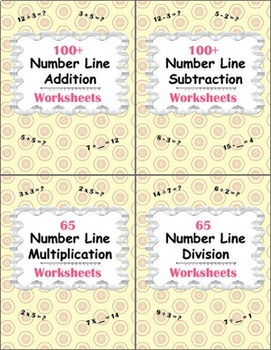Number Line Worksheets Bundle - Addition, Subtraction, Multiplication, Division\$12.00Number Line Bundle includes these products:
Number Line Subtraction Worksheets
Number Line Multiplication Worksheets
Number Line Division Worksheets
When purchased individually, the cost of these products totals \$16.00

Addition Using Number Line. Numbers 0 - 5. (25 Worksheets)
Addition Using Number Line. Numbers 0 - 10. (25 Worksheets)
Addition Using Number Line. Numbers 0 - 15. (25 Worksheets)

Subtraction
Subtraction Using Number Line. Numbers 0 - 5. (25 Worksheets)
Subtraction Using Number Line. Numbers 0 - 10. (25 Worksheets)
Subtraction Using Number Line. Numbers 0 - 15. (25 Worksheets)
Subtraction Using Number Line. Write the subtraction sentence. (5 Worksheets)
Subtraction Using Number Line. Missing Minuends/Subtrahends. (25 Worksheets)

Multiplication
Multiplication Using Number Line. Numbers 0 - 15. (30 Worksheets)
Multiplication Using Number Line. Write the multiplication sentence. (5 Worksheets)
Multiplication Using Number Line. Missing Multiplier. (30 Worksheets)

Division
Division Using Number Line. Numbers 0 - 15. (30 Worksheets)
Division Using Number Line. Write the division sentence. (5 Worksheets)
Division Using Number Line. Missing Divisor. (30 Worksheets)

----------------------------------

You May Also Like These Worksheets:
Number Line Subtraction Worksheets
Number Line Multiplication Worksheets
Number Line Division Worksheets
Number Line Worksheets Bundle [Bundle!]

You May Also Like These Task Cards: